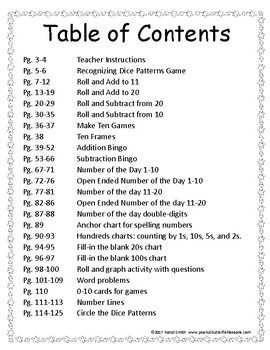DID YOU KNOW:
Seamlessly assign resources as digital activities

Learn how in 5 minutes with a tutorial resource. Try it Now

Learn More# Fall Number Sense Packet for K and 1st GradeK - 1st, Homeschool
Subjects
Standards
Resource Type
Formats Included
• PDF
Pages
191 pages

#### Also included in

1. These seasonally-themed number sense packets contain the materials, games, and worksheets you need to help your children build their early number sense. It will provide your children the opportunity to explore how numbers relate to each other and learn all of their addition and subtraction facts
\$20.00
\$24.00
Save \$4.00

### Description

This fall-themed number sense packet contains the materials, games, and worksheets you need to help your children build their early number sense. It will provide your children the opportunity to explore how numbers relate to each other and learn all of their addition and subtraction facts.

THE PACKET CONTAINS:

Subitizing worksheets and games.

Number sense manipulatives. Manipulatives include five and ten frames cards, larger 5 and 10 frames with manipulatives, number tiles, number path and part-part-whole mats.

Three levels of Number of the Day worksheets.

Hundreds charts for skip counting and filling in the blanks.

Games to teach how to derive addition and subtraction facts such as make 5 and 10, doubles addition and one and two more and less than.  Once children learn these facts, it is much easier to learn the others. Games include Memory, Go Fish, I Have Who Has, and Roll and Cover (aka Bump).

Games for practicing all addition and subtraction facts, including Roll and Cover (aka Bump), Bingo, and others.

Roll and graph activity with questions.

Story problems.

Children will enjoy the colorful pictures and learning math through hands on exploration and games.

Total Pages
191 pages
N/A
Teaching Duration
N/A
Report this Resource to TpT
Reported resources will be reviewed by our team. Report this resource to let us know if this resource violates TpT’s content guidelines.

### Standards

to see state-specific standards (only available in the US).
Add and subtract within 20, demonstrating fluency for addition and subtraction within 10. Use strategies such as counting on; making ten (e.g., 8 + 6 = 8 + 2 + 4 = 10 + 4 = 14); decomposing a number leading to a ten (e.g., 13 - 4 = 13 - 3 - 1 = 10 - 1 = 9); using the relationship between addition and subtraction (e.g., knowing that 8 + 4 = 12, one knows 12 - 8 = 4); and creating equivalent but easier or known sums (e.g., adding 6 + 7 by creating the known equivalent 6 + 6 + 1 = 12 + 1 = 13).
Use addition and subtraction within 20 to solve word problems involving situations of adding to, taking from, putting together, taking apart, and comparing, with unknowns in all positions, e.g., by using objects, drawings, and equations with a symbol for the unknown number to represent the problem.
Fluently add and subtract within 5.
Solve addition and subtraction word problems, and add and subtract within 10, e.g., by using objects or drawings to represent the problem.
Given a two-digit number, mentally find 10 more or 10 less than the number, without having to count; explain the reasoning used.